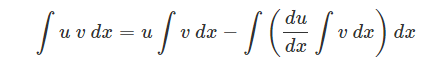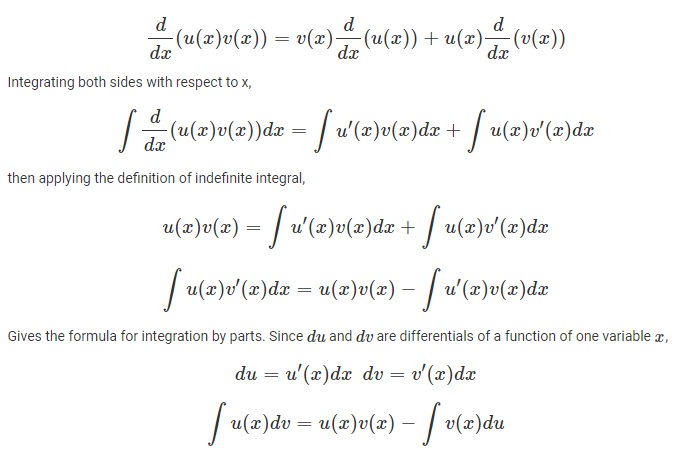# Integration By Parts Formula

Integration By Parts formula is used for integrating the product of two functions. This method is used to find the integrals by reducing them into standard forms. For example, if we have to find the integration of x sin x, then we need to use this formula. The integrand is the product of the two functions. The formula for integrating by parts is given by;Apart from integration by parts, there are two methods which are used to perform integration. They are:

• Integration by Substitution
• Integration using Partial Fractions

## Derivation of Integration By Parts Formula

If u(x) and v(x) are any two differentiable functions of a single variable y. Then, by the product rule of differentiation, we get;u’ is the derivative of u and v’ is the derivative of v.

To find the value of ∫vu′dx, we need to find the antiderivative of v’, present in the original integral ∫uv′dx.

Note:

• Integration by parts is not applicable for functions such as √x sin x dx.
• We do not add any constant while finding the integral of the second function.
• Usually, if any function is a power of x or a polynomial in x, then we take it as the first function. However, in cases where another function is an inverse trigonometric function or logarithmic function, then we take them as first function.

## Ilate Rule

In integration by parts, we have learned when the product of two functions are given to us then we apply the required formula. The integral of the two functions are taken, by considering the left term as first function and second term as the second function. This method is called Ilate rule. Suppose, we have to integrate x ex, then we consider x as first function and ex as the second function. So basically, the first function is chosen in such a way that the derivative of the function could be easily integrated. Usually, the preference order of this rule is based on some functions such as Inverse, Algebraic, Logarithm, Trigonometric, Exponent.

## Examples

Q.1: Find ∫ x cos x

Solution: Given, ∫ x cos x

The integrand here is the product of two functions.

Therefore, we have to apply the formula of integration by parts.

As per the formula, we have to consider, dv/dx as one function and u as another function.

Here, let x is equal to u, so that after differentiation, du/dx = 1, the value we get is a constant value.

Again,

u = x and dv/dx = cos x

We already found the value, du/dx = 1

Now, since dv/dx = cos x

On integrating both the sides we get;

v = ∫ cox dx

v = sin x

Now, using the formula,

∫u (dv/dx) dx = uv – ∫v (du/dx)dx

∫x cox x dx = x sin x – ∫ sin x . 1 dx

∫x cox x dx = x sinx + cos x + c

Where c is a constant.

Q.2: Find ∫log x dx

Solution: Let us assume here log x is the first function and constant 1 is the second function. Then the integral of the second function is x. Therefore,

∫(log x.1) dx = log x ∫1 dx – ∫[d/dx(log x) ∫1 dx]dx

= (log x).x – ∫1/x.x dx

= x log x – x + C

Q.3: Find ∫ ex sin x dx

Solution: Let us take ex as first function and sin x as the second function.

Now by integrating the functions by parts, we get;

I = ∫ ex sin x dx = ex (-cos x) + ∫ ex cos x dx

I = -ex cos x + I’ …..(1)

Now, taking ex as first function and cos x as second function, we get;

I’ = ex sin x – ∫ ex sin x dx

Putting the value of I’ in eq.(1), we get;

I = – ex cos x + ex sin x – I

Or

2I = ex (sin x – cos x)

Therefore, ∫ ex sin x dx = ex/2 (sin x – cos x) + C